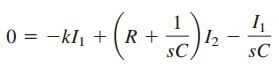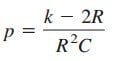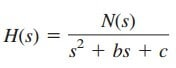# Short Example of Laplace Transform Network StabilityLike what we have studied before, the Laplace transform network stability is also one of the Laplace transform applications

## Laplace Transform Network Stability

A circuit is stable if its impulse response h(t) is bounded (i.e., h(t) converges to a finite value) as t → ∞; it is unstable if h(t) grows without bound as t → ∞. In mathematical terms, a circuit is stable when

Since the transfer function H(s) is the Laplace transform of the impulse response h(t), H(s) must meet certain requirements in order for Equation.(1) to hold. Recall that H(s) may be written as

where the roots of N(s) = 0 are called the zeros of H(s) because they make H(s) = 0, while the roots of D(s) = 0 are called the poles of H(s) since they cause H(s) → ∞.

The zeros and poles of H(s) are often located in the s plane as shown in Figure.(1a). Recall from Equations.(1) and (2) from the Laplace transform inverse, that H(s) may also be written in terms of its poles as

H(s) must meet two requirements for the circuit to be stable. First, the degree of N(s) must be less than the degree of D(s); otherwise, the long division would produce

where the degree of R(s), the remainder of the long division, is less than the degree of D(s). The inverse of H(s) in Equation.(4) does not meet the condition in Equation.(1).

Second, all the poles of H(s) in Equation.(2) (i.e., all the roots of D(s) = 0) must have negative real parts; in other words, all the poles must lie in the left half of the s plane, as shown typically in Figure.(1b).

The reason for this will be apparent if we take the inverse Laplace transform of H(s) in Equation.(2). Because Equation.(2) is similar to Equation.(3), its partial fraction expansion is similar to the one in Laplace transform inverse so that the inverse of H(s) is similar to that in Equation.(7) in the Laplace transform inverse. Hence,

We see from this equation that each pole pi must be positive
(i.e., pole
s = –pi in the left-half plane)
for epit to decrease with increasing t. Thus,

A circuit is stable when all the poles of its transfer function H(s) lie in the left half of the s plane.

An unstable circuit never reaches a steady state because the transient response does not decay to zero. Consequently, steady-state analysis is only applicable to stable circuits.

A circuit made up exclusively of passive elements (R, L, and C) and independent sources cannot be unstable, because that would imply that some branch currents or voltages would grow indefinitely with sources set to zero. Passive elements cannot generate such indefinite growth.

Passive circuits either are stable or have poles with zero real parts. To show that this is the case, consider the series RLC circuit in Figure.(2).

The transfer function is given by

Notice that D(s) = s2 + sRL + 1LC = 0 is the same as the characteristic equation obtained for the series RLC circuit. The circuit has poles at

For R, L, C > 0, the two poles always lie in the left half of the s plane, implying that the circuit is always stable. However, when R = 0, α = 0 and the circuit becomes unstable. Although ideally, this is possible, it does not really happen, because R is never zero.

On the other hand, active circuits or passive circuits with controlled sources can supply energy, and they can be unstable. In fact, an oscillator is a typical example of a circuit designed to be unstable. An oscillator is designed such that its transfer function is of the form

so that its output is sinusoidal.

## Laplace Transform Network Stability Examples

1. Determine the values of k for which the circuit in Figure.(3) is stable.

Solution:
Applying mesh analysis to the first-order circuit in Figure.(3) gives

andor

We can write Equations.(1.1) and (1.2) in matrix form asThe determinant is

The characteristic equation (∆ = 0) gives the single-pole aswhich is negative when k < 2R. Thus, we conclude the circuit is stable when k < 2R and unstable for k > 2R.

2. An active filter has the transfer functionFor what values of k is the filter stable?

Solution:
As a second-order circuit, H(s) may be written aswhere b = 4 − k, c = 1, and N(s) = k.
This has poles at p2 + bp + c = 0; that is,For the circuit to be stable, the poles must be located in the left half of the s plane. This implies that b > 0.

Applying this to the given H(s) means that for the circuit to be stable,
4 − k > 0 or k < 4.